# Two-Dimensional True Compression

Starting from a given image, the goal of true compression is to minimize the number of bits needed to represent it, while storing information of acceptable quality. Wavelets contribute to effective solutions for this problem. The complete chain of compression includes iterative phases of quantization, coding, and decoding, in addition to the wavelet processing itself.

The purpose of this example is to show how to decompose, compress, and decompress a grayscale or truecolor image using various compression methods. To illustrate these capabilities, we consider a grayscale image of a mask and a truecolor image of peppers.

### Compression by Global Thresholding and Huffman Encoding

```load mask image(X) axis square colormap(pink(255)) title('Original Image: mask')```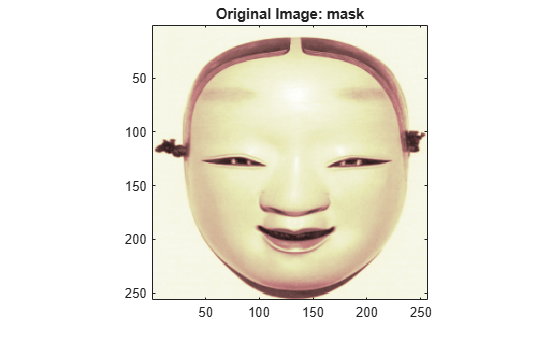A measure of achieved compression is given by the compression ratio (CR) and the Bit-Per-Pixel (BPP) ratio. CR and BPP represent equivalent information. CR indicates that the compressed image is stored using CR % of the initial storage size while BPP is the number of bits used to store one pixel of the image. For a grayscale image the initial BPP is 8. For a truecolor image the initial BPP is 24, because 8 bits are used to encode each of the three colors (RGB color space).

The challenge of compression methods is to find the best compromise between a low compression ratio and a good perceptual result.

We begin with a simple method of cascading global coefficients thresholding and Huffman encoding. We use the default wavelet bior4.4 and the default level, which is the maximum possible level (see the `WMAXLEV` function) divided by 2. The desired BPP is set to 0.5 and the compressed image is stored in the file named mask.wtc.

```meth = 'gbl_mmc_h'; % Method name option = 'c'; % 'c' stands for compression [CR,BPP] = wcompress(option,X,'mask.wtc',meth,'BPP',0.5)```
```CR = 6.7200 ```
```BPP = 0.5376 ```

The achieved Bit-Per-Pixel ratio is actually about 0.53 (closed to the desired one of 0.5) for a compression ratio of 6.7%.

### Uncompression

Now we decompress the image retrieved from the file mask.wtc and compare it to the original image.

```option = 'u'; % 'u' stands for uncompression Xc = wcompress(option,'mask.wtc'); colormap(pink(255))``````subplot(1,2,1); image(X); axis square; title('Original Image') subplot(1,2,2); image(Xc); axis square; title('Compressed Image') xlabel({['Compression Ratio: ' num2str(CR,'%1.2f %%')], ... ['BPP: ' num2str(BPP,'%3.2f')]})```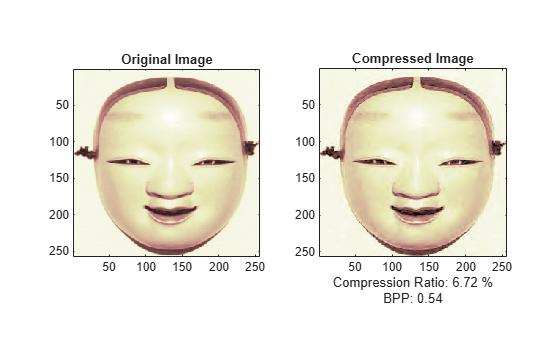The result is satisfactory, but a better compromise between compression ratio and visual quality can be obtained using more sophisticated true compression methods, which involve tighter thresholding and quantization steps.

### Compression by Progressive Methods

We now illustrate the use of progressive methods of compression, starting with the EZW algorithm using the Haar wavelet. The key parameter is the number of loops; increasing it leads to better recovery, but a worse compression ratio.

```meth = 'ezw'; % Method name wname = 'haar'; % Wavelet name nbloop = 6; % Number of loops [CR,BPP] = wcompress('c',X,'mask.wtc',meth,'maxloop', nbloop, ... 'wname','haar'); Xc = wcompress('u','mask.wtc'); colormap(pink(255)) subplot(1,2,1); image(X); axis square; title('Original Image') subplot(1,2,2); image(Xc); axis square; title('Compressed Image - 6 steps') xlabel({['Compression Ratio: ' num2str(CR,'%1.2f %%')], ... ['BPP: ' num2str(BPP,'%3.2f')]})```Here, using only 6 steps produces a very coarse decompressed image. Now we examine a slightly better result using 9 steps and finally a satisfactory result using 12 steps.

```[CR,BPP] = wcompress('c',X,'mask.wtc',meth,'maxloop',9,'wname','haar'); Xc = wcompress('u','mask.wtc'); colormap(pink(255)) subplot(1,2,1); image(Xc); axis square; title('Compressed Image - 9 steps') xlabel({['Compression Ratio: ' num2str(CR,'%1.2f %%')],... ['BPP: ' num2str(BPP,'%3.2f')]}) [CR,BPP] = wcompress('c',X,'mask.wtc',meth,'maxloop',12,'wname','haar'); Xc = wcompress('u','mask.wtc'); subplot(1,2,2); image(Xc); axis square; title('Compressed Image - 12 steps') xlabel({['Compression Ratio: ' num2str(CR,'%1.2f %%')], ... ['BPP: ' num2str(BPP,'%3.2f')]})```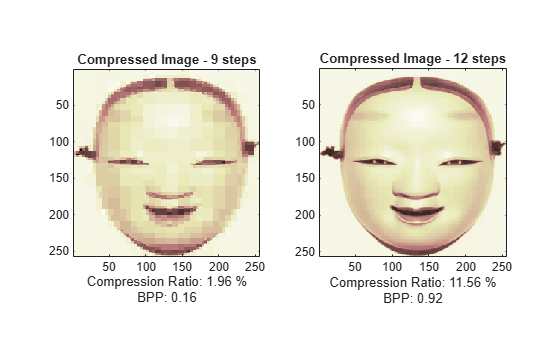The final BPP ratio is approximately 0.92 when using 12 steps.

Now we try to improve the results by using the wavelet bior4.4 instead of haar and looking at loops of 12 and 11 steps.

```[CR,BPP] = wcompress('c',X,'mask.wtc','ezw','maxloop',12, ... 'wname','bior4.4'); Xc = wcompress('u','mask.wtc'); colormap(pink(255)) subplot(1,2,1); image(Xc); axis square; title('Compressed Image - 12 steps') xlabel({['Compression Ratio: ' num2str(CR,'%1.2f %%')], ... ['BPP: ' num2str(BPP,'%3.2f')]}) [CR,BPP] = wcompress('c',X,'mask.wtc','ezw','maxloop',11, ... 'wname','bior4.4'); Xc = wcompress('u','mask.wtc'); subplot(1,2,2); image(Xc); axis square; title('Compressed Image - 11 steps') xlabel({['Compression Ratio: ' num2str(CR,'%1.2f %%')], ... ['BPP: ' num2str(BPP,'%3.2f')]})```For the eleventh loop, we see that the result can be considered satisfactory, and the obtained BPP ratio is approximately 0.35. By using a more recent method, SPIHT (Set Partitioning in Hierarchical Trees), the BPP can be improved further.

```[CR,BPP] = wcompress('c',X,'mask.wtc','spiht','maxloop',12, ... 'wname','bior4.4'); Xc = wcompress('u','mask.wtc'); colormap(pink(255)) subplot(1,2,1); image(X); axis square; title('Original Image') subplot(1,2,2); image(Xc); axis square; title('Compressed Image - 12 steps') xlabel({['Compression Ratio: ' num2str(CR,'%1.2f %%')], ... ['BPP: ' num2str(BPP,'%3.2f')]})```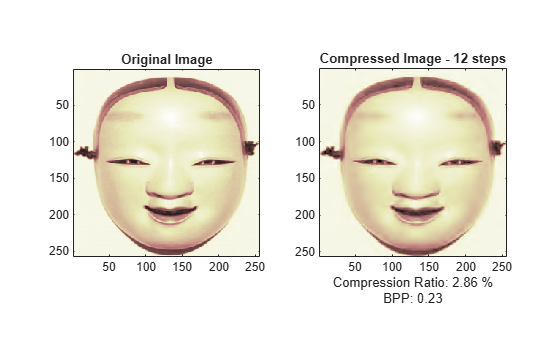The final compression ratio (2.8%) and Bit-Per-Pixel ratio (0.23) are very satisfactory. Recall that the CR means that the compressed image is stored using only 2.8% of the initial storage size.

### Handling Truecolor Images

Finally, we illustrate how to compress the wpeppers.jpg truecolor image. Truecolor images can be compressed using the same scheme as grayscale images by applying the same strategies to each of the three color components.

The progressive compression method used is SPIHT (Set Partitioning in Hierarchical Trees) and the number of encoding loops is set to 12.

```X = imread('wpeppers.jpg'); [CR,BPP] = wcompress('c',X,'wpeppers.wtc','spiht','maxloop',12); Xc = wcompress('u','wpeppers.wtc'); colormap(pink(255)) subplot(1,2,1); image(X); axis square; title('Original Image') subplot(1,2,2); image(Xc); axis square; title('Compressed Image - 12 steps') xlabel({['Compression Ratio: ' num2str(CR,'%1.2f %%')], ... ['BPP: ' num2str(BPP,'%3.2f')]})```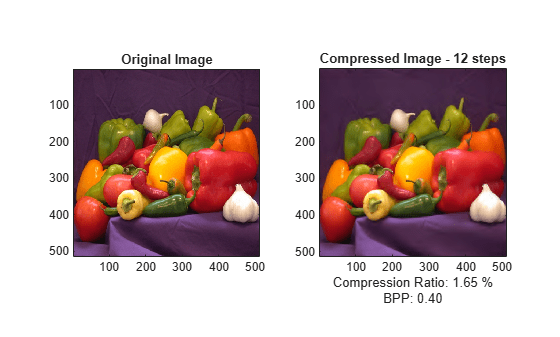`delete('wpeppers.wtc')`

The compression ratio (1.65%) and the Bit-Per-Pixel ratio (0.4) are very satisfactory while maintaining a good visual perception.

### More about True Compression of Images

For more information about True Compression of images, including some theory and examples, see the following reference:

Misiti, M., Y. Misiti, G. Oppenheim, J.-M. Poggi (2007), "Wavelets and their applications", ISTE DSP Series.

##### SupportGet trial now